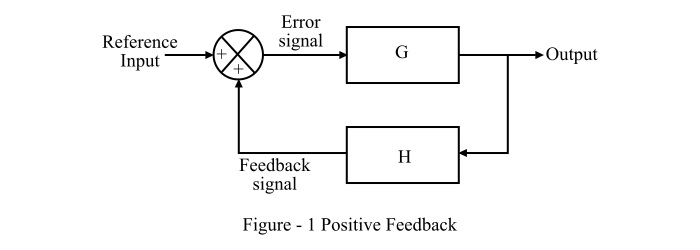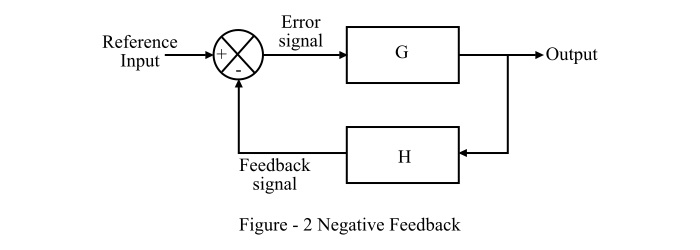# Difference between Positive and Negative Feedback in a Control System

In a control system, the process of sampling and returning the output (or a part of output) to the input end of the system is known as feedback. The feedback is used to improve the operating performance to obtain the desired output from a control system. The feedback signal may be a voltage or a current depending on the operation of the feedback sampling system. It must remember that the feedback is a unidirectional process, i.e. the sampled feedback signal always flows from output to input side. The use of feedback in a system makes it a closed loop control system.

Therefore, the feedback plays a vital role in any control system as it improves the system performance and makes the system to produce more accurate and reliable output. Hence, feedback is extensively used in the several applications like in process control systems, oscillators, amplifiers, traffic control system, etc.

The feedback used in the control systems are majorly classified into two types namely,

• Positive Feedback
• Negative Feedback

Read through this article to understand the major differences between positive feedback and negative feedback in a control system. But before discussing the differences, let's start with some basics of positive feedback and negative feedback.

## What is Positive Feedback?

The type of feedback in a control system which samples the output signal and fed it to the input in phase with the applied input signal us called positive feedback. In simple words, a feedback in which the reference input signal and the feedback signal are added together is called a positive feedback. The positive feedback is also called a regenerative feedback. Figure-1 shows the block diagram of positive feedback in a control system.The transfer function of a control system with positive feedback is given by the following expression,

$$TF\:=\:\frac{G}{1\:-\:GH}$$

Where, TF is the transfer function of system, G is the open loop gain, and H is the gain feedback path.

The positive feedback, therefore, increases the gain of the control system. Consequently, the one major application of positive feedback is in oscillator circuits.

## What is Negative Feedback?

The type of feedback in a control system in which the feedback signal and the reference input signal are out of phase is called a negative feedback. In simple words, when the reference input signal and the feedback signal are subtracted at the input of the system, then the feedback is known as negative feedback. The negative feedback is also known as degenerative feedback.

Therefore, in case of negative feedback, the difference of input signal and feedback signal drives the system. This difference is known as error signal. Due to several technical benefits, the negative feedback is the most widely used type of feedback in the control systems. Figure-2 shows the block diagram of the negative feedback.The transfer function of a control system having negative feedback is given by the following expression,

$$TF\:=\:\frac{G}{1\:+\:GH}$$

As in the negative feedback, the error signal is a difference of two signal. Therefore, the gain of the control system with the negative feedback is low. The negative feedback is mostly used in the amplifiers.

## Difference between Positive Feedback and Negative Feedback

The following table highlights all the significant differences between positive feedback and negative feedback in a control system −

Basis of Difference Positive Feedback Negative Feedback
Definition The type of feedback in which the feedback signal and the reference input signal are added at the input side of the system to produce the error signal is called a positive feedback. The type of feedback in which the feedback signal and the reference input signal are subtracted at the input side of the system to produce the error signal is known as negative feedback.
Alternate name The positive feedback is also called a regenerative feedback. The negative feedback is also called a degenerative feedback.
Error signal Error signal = Reference input signal + feedback signal. Hence, the positive feedback produces an increased error signal. Error signal = Reference input signal - feedback signal. Hence, the negative feedback produces a decreased error signal.
Transfer function

The closed loop transfer function of a control system with positive feedback is given by,

$$TF\:=\:\frac{G}{1\:-\:GH}$$

The closed loop transfer function of a control system with negative feedback is given by,

$$TF\:=\:\frac{G}{1\:+\:GH}$$

System gain The gain of the control system with positive feedback is high. The gain of the control system with negative feedback is low.
System stability The stability of a control system having positive feedback is low. The stability of a system having negative feedback is high.
Phase relation between input and feedback signals In case of positive feedback, the reference input and feedback signals are in phase (i.e. 0° phase difference). In case of negative feedback, the reference input signal and the feedback signal are out of phase (i.e. 180° phase difference).
Sensitivity to change in parameters Positive feedback is highly sensitive to the change in system parameters. In case of negative feedback, the sensitivity to the parameter changes is low.
Feedback in Op - Amp circuits The non-inverting (positive) terminal of an Op-Amp (operational amplifier) is used to provide positive feedback. The inverting (negative) terminal of Op-Amp is used to provide the negative feedback.
Overall gain The overall gain of the system with positive feedback is greater than the gain without feedback. The overall gain of the system with negative feedback is less than the gain without feedback.
Applications The positive feedback is extensively used in the oscillator circuits. The negative feedback is used in mostly amplifier circuits and many other control systems like motor control system, etc.

## Conclusion

The most significant difference is that in case of positive feedback, the error signal is the sum of input and feedback signals; whereas in case of negative feedback system, the error signal is the difference of input signal and feedback signal. In practical control systems, the negative feedback has more applications than positive feedback.

Updated on: 02-Sep-2022

14K+ Views